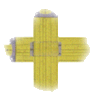#Entisoft Units Source Code Sample

This is a sample of the Visual Basic source code that you will receive when you register Entisoft Units.

```Function estConvertFindCat (ByVal vvarUnits As Variant) As Variant
' Return the name of the most common physical measurement described by the measurement expression.
' For example, the expression "meters/seconds" (and any other expression which involves a combination of length over
' time) is most commonly used to represent measurements of velocity.

On Error GoTo Error_estConvertFindCat

' Display the opening nag screen in the when this conversion function is first used within Shareware versions of the library.
If estSharewarVersion Then
MsgBox "Thank you for trying the Shareware version of Entisoft Units.  If you find this program useful and you continue to use it past the 30 day trial period, you are obligated to register it for \$39.95.  Registered users receive complete source code, additional program versions, additional conversion factors definitions, and technical support.  Please see the About box for ordering information.  Thanks for your support.", estvbInformation + estvbSystemModal, "Entisoft Units Shareware UNREGISTERED"
End If
End If

' Return Null when the argument is Null.
If Not estFixUpVariantToStringFn(vvarUnits) Then
estConvertFindCat = Null
Exit Function
End If

' Initialize the measurement conversion system if necessary.
Call estConvertInitMaybe

' This function converts the definition of each Category-type unit into base units then caches (stores) that to
' speed future calls to this function.
If Not estConvParDefDone Then
' Clear the vector which stores the hashed value of the converted definition strings.
ReDim estConvParDefHash(0 To estConvParDefHashSize)

Dim avarFm() As Variant
Dim varToBaseRtn As Variant
Dim dblConst As Double
Dim dblSub As Double

' Go through all of the Category-type unit definitions.
Dim intCurUnit As Integer
For intCurUnit = 1 To estConvNumUnit
If StrComp(Trim\$(estConvUnit(intCurUnit).sType), estConvertTypeCategory, estCompareText) = 0 Then
' Get the definition of the current Category-type unit.
Dim strDef As String
strDef = Trim\$(estConvUnit(intCurUnit).sDef)

' Convert the category definition to base units.
ReDim avarFm(0 To 2, 0 To 32)
dblConst = 0
varToBaseRtn = estConvertToBaseUnits(avarFm(), dblConst, ByVal strDef, dblSub, ByVal True, ByVal False)
If VarType(varToBaseRtn) = estvbString Then
' Lower-level errors in the measurement expression are echoed to the calling routine.
estConvertFindCat = varToBaseRtn
Exit Function
End If

' Need to sort the base units in the measurement expression so that any user-defined order of operands
' within the definitions does not affect this function which must find matching combinations of units
' quickly.

' Store the definition of the category in terms of base units.
Dim strParDef As String
strParDef = estConvertUnitsToStringSorted(avarFm())
estConvUnit(intCurUnit).sParDef = strParDef

' Insert or update an entry within the category definitions hash table.
Dim intHashPos As Integer
intHashPos = estConvertHashSelectParDef(strParDef)
estConvParDefHash(intHashPos) = intCurUnit
End If
Next intCurUnit

' Record that this initialization has been completed.
estConvParDefDone = True
End If

' Convert measurement to base units, returning the result when there is an error.
ReDim avarFm(0 To 2, 0 To 32)
dblConst = 0
varToBaseRtn = estConvertToBaseUnits(avarFm(), dblConst, ByVal vvarUnits, dblSub, ByVal True, ByVal False)

If VarType(varToBaseRtn) = estvbString Then
estConvertFindCat = varToBaseRtn
GoTo End_estConvertFindCat
End If

' Look for the same combination of base units among the category definitions.
Dim intUnit As Integer
strParDef = estConvertUnitsToStringSorted(avarFm())
intUnit = estConvParDefHash(estConvertHashSelectParDef(strParDef))
If intUnit <> 0 Then
' There was a category whose definition contains the same combination of base units as the argument to this function.
estConvertFindCat = Trim\$(estConvUnit(intUnit).sCode)
GoTo End_estConvertFindCat
End If

' Since no matching category was found, form the reciprocal of the measurement expression and see if its category is known.
' Form the reciprocal of each of the base units.
For intCurUnit = 1 To UBound(avarFm, 2)
avarFm(estConvExpCol, intCurUnit) = -avarFm(estConvExpCol, intCurUnit)
Next intCurUnit

' Look for the category of the reciprocal of the units.
strParDef = estConvertUnitsToStringSorted(avarFm())
intUnit = estConvParDefHash(estConvertHashSelectParDef(strParDef))
If intUnit <> 0 Then
' A category was found.
estConvertFindCat = Trim\$("reciprocal of " + estConvUnit(intUnit).sCode)
GoTo End_estConvertFindCat
End If

' No category could be found; return "unknown"
estConvertFindCat = "unknown"

End_estConvertFindCat:
Exit Function

Error_estConvertFindCat:
estConvertFindCat = "Warning: I have encountered error #" & Err & " (" & Error\$ & ") while performing the conversion."
Resume End_estConvertFindCat
End Function```